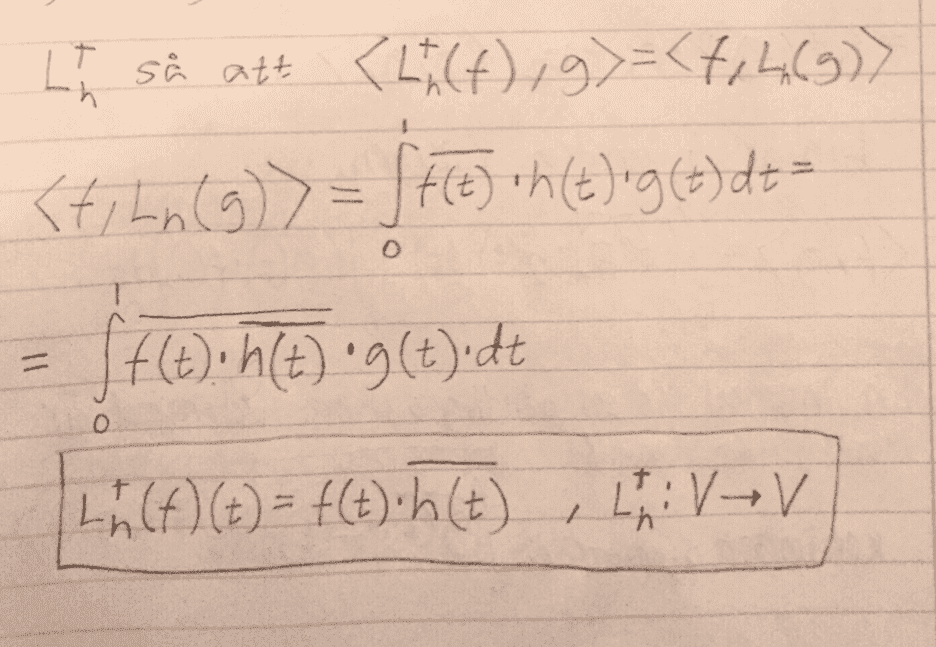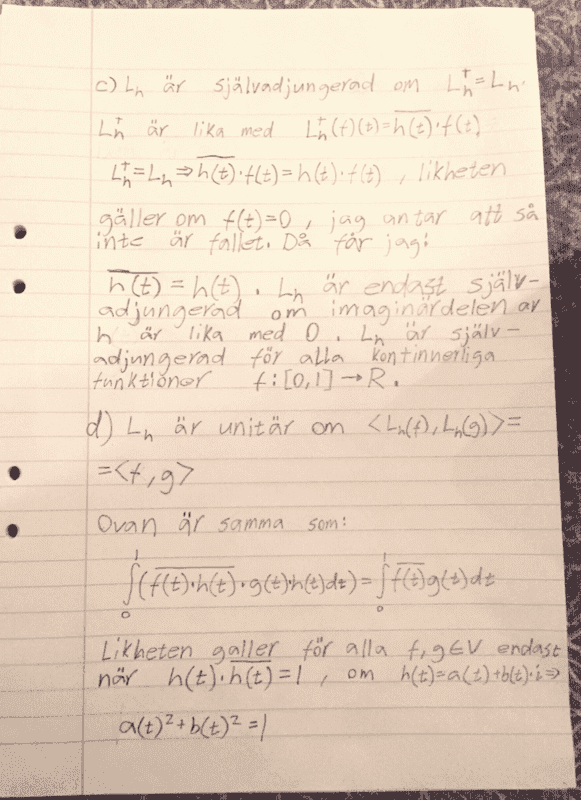# Linear algebra inner products, self adjoint operator,unitary operation

Karl Karlsson
Homework Statement:
Let ##V = \mathbb{C}([0, 1], \mathbb{C})## be the vector space of continuous functions ##f: [0,1] \rightarrow \mathbb{C}## with inner product:
$$\langle f|g \rangle = \int_0^1 \overline {f(t)}g(t)\;\mathrm{d}t$$

For ##h \in V## , let ##L_h: V \rightarrow V## be the operator defined by ##L_h(f)(t)=h(t)f(t)##.
b) Determine the adjoint operator to ##L_h##
c) For which functions is the operator ##L_h## self adjoint?
d) For which functions is the operator ##L_h## unitary?
e) Give example of one function h(t) such that the operator ##L_h## is self adjoint and has at least two different eigenvalues
Relevant Equations:
$$\langle f|g \rangle = \int_0^1 \overline {f(t)}g(t)\;\mathrm{d}t$$
##L_h(f)(t)=h(t)f(t)##
b)c and d):
In c) I say that ##L_h## is only self adjoint if the imaginary part of h is 0, is this correct?e) Here I could only come up with eigenvalues when h is some constant say C, then C is an eigenvalue. But I' can't find two.

Otherwise does b-d above look correct?

•Delta2

Homework Helper
Gold Member
2022 Award
It's looks all right. For d) perhaps think about writing ##h(t)## in polar form - that might be neater.

For e), remember these functions are only continuous. You have lots of options for where they are zero and non-zero.

Karl Karlsson
It's looks all right. For d) perhaps think about writing ##h(t)## in polar form - that might be neater.
Do you mean having ##a(t)=r\cdot cos(t)## and ##b(t)=r\cdot sin(t)## and r=1 ?
For e), remember these functions are only continuous. You have lots of options for where they are zero and non-zero.
Like what? I can't see how it would work for cos(t) ##e^t## t or any other normal continuous function

Homework Helper
Gold Member
2022 Award
Do you mean having ##a(t)=r\cdot cos(t)## and ##b(t)=r\cdot sin(t)## and r=1 ?

Like what? I can't see how it would work for cos(t) ##e^t## t or any other normal continuous function

I was thinking ##e^{ia(t)}## for some real function ##a(t)##. But, what you have is fine.

Try thinking about continuous functions more generally. Something like ##\cos t## is no good. That would never work. What's "normal"?

Karl Karlsson
Try thinking about continuous functions more generally. Something like ##\cos t## is no good. That would never work. What's "normal"?
I was just mentioning som elementary continuous functions that came to my mind but none of those seem to work and I can't find one that does.

Homework Helper
Gold Member
2022 Award
I was just mentioning som elementary continuous functions that came to my mind but none of those seem to work and I can't find one that does.

Karl Karlsson
Ooh, right! You mean if I for example said h(t)=1 in the intervall 0<t<0.5 and h(t)=2 in the intervall 0.5<t<1. One eigenvector for the eigenvalue 1 could be the function f(t) which is ##t-0.5## on the intervall 0<t<0.5 and f(t)=0 on 0.5<t<1 ? And for for the eigenvalue 2 an eigenvector could be g(t) = 0 on 0<t<0.5 and ##g(t) =t-0.5## on 0.5<t<1?

Homework Helper
Gold Member
2022 Award
Ooh, right! You mean if I for example said h(t)=1 in the intervall 0<t<0.5 and h(t)=2 in the intervall 0.5<t<1. One eigenvector for the eigenvalue 1 could be the function f(t) which is ##t-0.5## on the intervall 0<t<0.5 and f(t)=0 on 0.5<t<1 ? And for for the eigenvalue 2 an eigenvector could be g(t) = 0 on 0<t<0.5 and ##g(t) =t-0.5## on 0.5<t<1?
That's not a continuous step function. You need to join the steps. The eigenfunctions would be any function that is zero outside one of the steps.

Karl Karlsson
That's not a continuous step function. You need to join the steps. The eigenfunctions would be any function that is zero outside one of the steps.
Yeah, I realized that afterwards. So i can choose h = 1 on 0 <= t <= 1/3 and h=3t on 1/3 < t < 2/3 and h=2 on 2/3 <=t <= 1. An eigenvector to eigenvalue 1 could be a function that is t-1/3 on the intervall 0<=t<=1/3 and 0 on 1/3<t<=1. An eigenvector to eigenvalue 2 could be a function that is 0 on the interval 0<=t<2/3 and t-2/3 on 2/3<=t<=1. Is this correct?

•PeroK